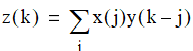# NMath User's Guide

10.2 Convolution and Correlation (.NET, C#, CSharp, VB, Visual Basic, F#)

Convolution is used to linearly filter a signal The convolution z(n) of two discrete input sequences x(n) and y(n) is defined as:Mathematically, the two convolved vectors, x and y, can be interchanged without changing the convolution result, z. In practice, however, one vector, called the convolution kernel, is often much shorter than the other and is typically used in many convolution operations against different data sets. The kernel can be thought of as a moving window scanned across the data vector. The output value is the weighted sum of the data within the window multiplied by the kernel. Where necessary, the sum is computed by padding the edges of the data with zeros. If the data is of length m and the kernel is of length n, then the output is of length m+n-1.

Correlation is used to characterize the statistical similarity between two signals. The operation is very similar to convolution, in that correlation uses two signals to produce a third signal, called the cross-correlation, or, if a signal is correlated with itself, the autocorrelation. The correlation is defined as:NMath provides classes for performing linear convolutions on real and complex 1D data. The API is

Convolution and Correlation Classes

The classes that perform 1D convolution and correlation in NMath are named <Type>1DConvolution and <Type>1DCorrelation, respectively, where <Type> is Float, Double, FloatComplex, or DoubleComplex. For example, class performs convolutions of two 1D sequences of double-precision floating point values.

Creating Convolution and Correlation Instances

Convolution and correlation instances are constructed by specifying the kernel and the length of the data vector. For example, this code constructs a Double1DConvolution for a kernel of length 5, representing a moving average, and data vector of length 1024:

Code Example – C# FFT

```var kernel = new DoubleVector( ".2 .2 .2 .2 .2" );
```
```int dataLength = 1024;
```
```Double1DConvolution conv =
```
```  new Double1DConvolution( kernel, dataLength );
```

Code Example – VB FFT

```Dim Kernel As New DoubleVector(".2 .2 .2 .2 .2")
```
```Dim DataLength = 1024
```
```Dim Conv As New Double1DConvolution(Kernel, DataLength)
```

The kernel can be supplied either using an NMath vector or an array. For an NMath vector, a kernel offset and stride can be specified on the vector instance. For an array, a separate integer kernel offset and stride may be passed to the constructor:

Code Example – C# FFT

```var kernel = new DoubleVector( "-1 .2 -1 .2 -1 .2" );
```
```int kernelOffset = 1;
```
```int kernelStride = 2;
```
```int dataLength = 1024;
```
```var corr = new Double1DCorrelation( kernel, kernelOffset,
```
```  kernelStride, dataLength );
```

Code Example – VB FFT

```Dim Kernel As New DoubleVector("-1 .2 -1 .2 -1 .2")
```
```Dim KernelOffset = 1
```
```Dim KernelStride = 2
```
```Dim DataLength = 1024
```
```Dim Corr As New Double1DCorrelation(Kernel, KernelOffset,
```
```  KernelStride, DataLength)
```

Convolution and Correlation Properties

Once constructed, an NMath convolution or correlation object provides the following read-only properties:

KernelLength gets the length of the kernel.

DataLength gets the expected convolution or correlation data length.

Length gets the length of the output convolution or correlation. The output length equals DataLength + KernelLength - 1.

Computing Convolutions and Correlations

The Convolve() method computes the convolution and the Correlate() method computes the correlation between the stored kernel, and a given data vector. For example:

Code Example – C# FFT

```var data = new FloatVector( 500, new RandGenUniform() );
```
```FloatVector result = corr.Correlate( data );
```

Code Example – VB FFT

```Dim Data As New FloatVector(500, New RandGenUniform())
```
```Dim Result As FloatVector = Corr.Correlate(Data)
```

An InvalidArgumentException is raised if the length of the given data does not match the data length previously specified in the constructor.

If you are performing multiple convolutions or correlations using the same object—within a loop, for example—you can reuse the same pre-allocated vector to hold the result:

Code Example – C# FFT

```var data = new FloatVector( 500, new RandGenUniform() );
```
```var result = new FloatVector( corr.Length );
```
```corr.Correlate( data, ref result );
```

Code Example – VB FFT

```Dim Data As New FloatVector(500, New RandGenUniform())
```
```Dim Result As New FloatVector(Corr.Length)
```
```Corr.Correlate(Data, Result)
```

Windowing Options

The Convolve() and Correlate() methods compute the full result, with length DataLength + KernelLength - 1. Boundary values, where the kernel partially overlaps the data, are computed by padding the edges of the data with zeros. The TrimConvolution() and TrimCorrelation() methods creates a clipped view into a given result, using the specified Windowing option:

Windowing.Unwindowed (the default) retrieves the full result.

Windowing.CenterWindow clips the result to the length of the data, shifted to the center.

Windowing.FullKernelOverlap returns the data portion that entirely overlaps the kernel.

For instance:

Code Example – C# FFT

```DoubleVector result = conv.Convolve( data );
```
```DoubleVector trimmed = conv.TrimConvolution( result,
```
```  CorrelationBase.Windowing.FullKernelOverlap );
```

Code Example – VB FFT

```Dim Result As DoubleVector = Conv.Convolve(Data)
```
```Dim Trimmed As DoubleVector = Conv.TrimConvolution(result,
```
```  CorrelationBase.Windowing.FullKernelOverlap)
```

No data is copied. The returned vector is a view into the same data referenced by the given result.

Top

Top